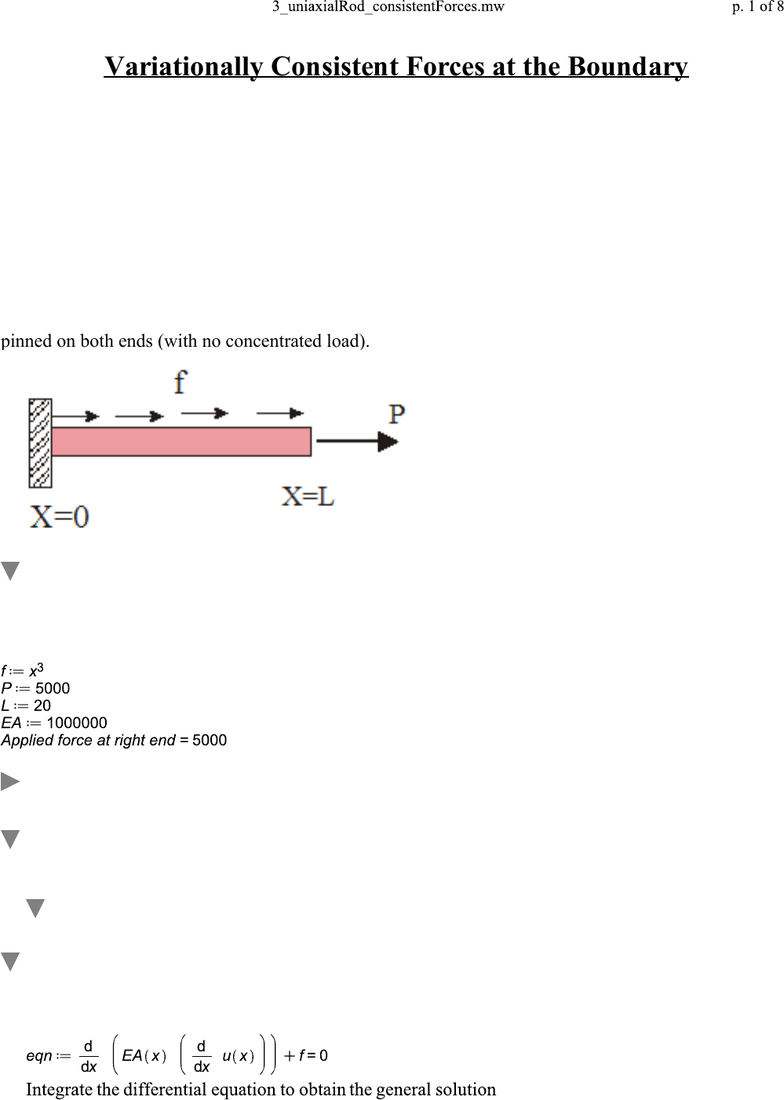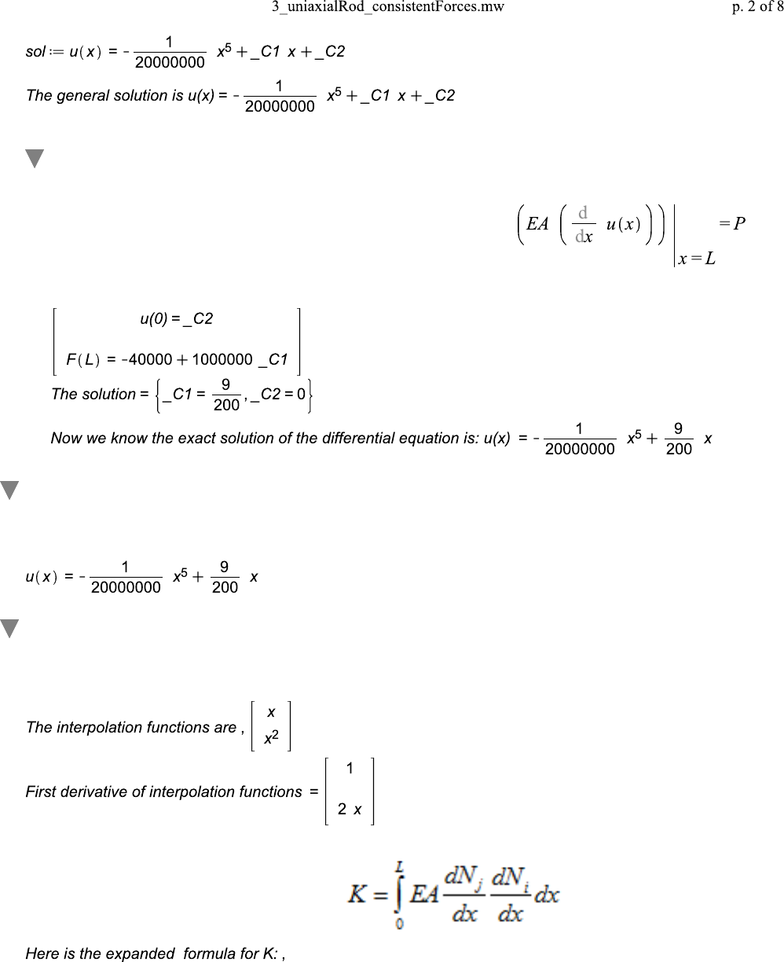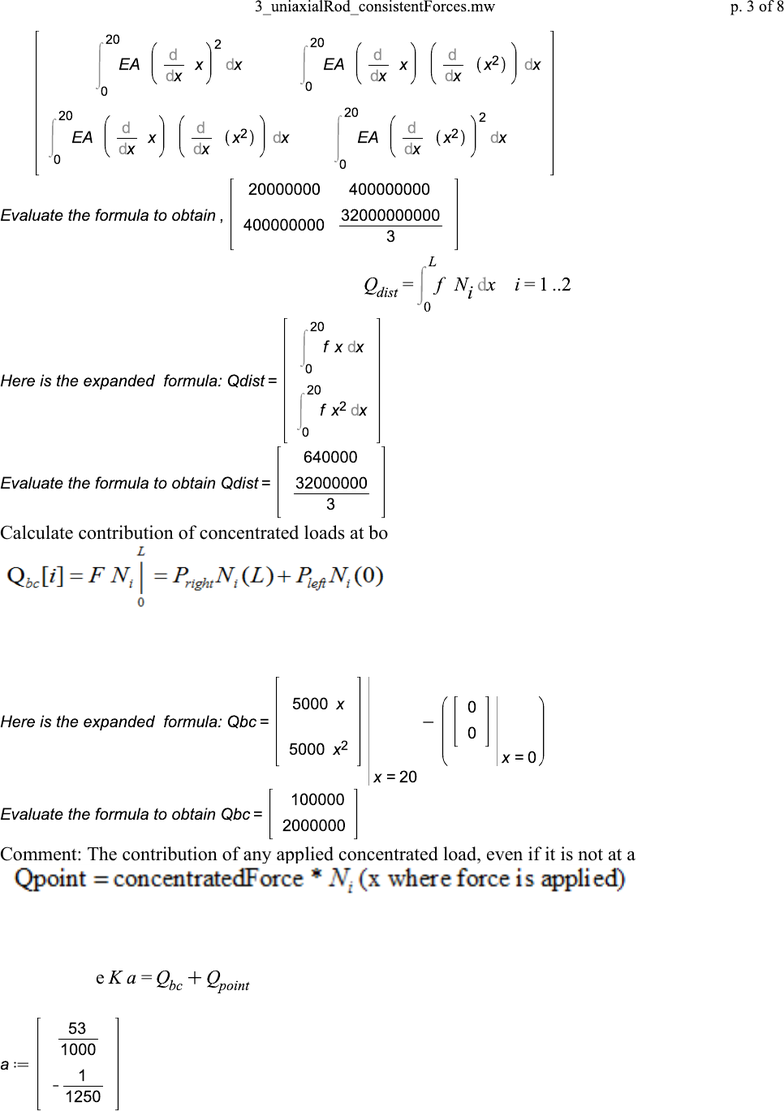Study Guides (400,000)
US (230,000)
TAMU (3,000)
AERO (10)
Final

# AERO 306 Lecture 13: 3_uniaxialRod_consistentForcesExam

Department
Aerospace Engineering
Course Code
AERO 306
Professor
John Whitcomb
Study Guide
Final

This preview shows pages 1-2. to view the full 8 pages of the document.(4.1)(4.1)
(1)(1)
Variationally Consistent Forces at the Boundary
"The accurate way to calculate the boundary forces"
The example will demonstrate a technique for calculating the forces acting at the boundaries where
kinematic constraints are applied. This technique is more accurate than using the approximate solution to
calculate the strain, etc. This technique will be referred to as the "variationally consistent" method.
The variationally consistent method can be used without the matrix formulas, but we will not cover it in
class.
After going through this problem, you should solve a problem in which the boundary conditions are
different, such a one that is pinned only on the right end (with a concentrated load on the left end) or
pinned on both ends (with no concentrated load).
Before getting into the Maple calculations...
Before getting into the details for a 2-term solution, which was solved using Maple, we will do a
1-term solution by hand. If I forget in class... remind me!
Utilities
Summary of equations
Matrix formulas
Exact solution of differential equation... like you do by hand
We will use this for evaluating the accuracy of our approximate solution.
The equilibrium equation written in terms of displacement is

Only pages 1-2 are available for preview. Some parts have been intentionally blurred.(6.1)(6.1)
(4.2)(4.2)
(4.1.1)(4.1.1)
(5.1)(5.1)
(6.2)(6.2)
Impose BC's to determine integration constants _C1 and _C2
Note that the BC's must be expresssed in terms of the general solution for u(x).
The boundary conditions for this problem are: u(0)=0 and
Solve the simultaneous equations: u(0)=0 and applied force at L =P.
In terms of the general solution, these equations are
Exact solution of differential equation using more Maple
"power"
Now let's calculate a 2-Term approximate solution
The interpolation functions are obtained by first obtaining a valid assumed solution and then
picking out whatever multiplies the unknowns.
The formula for the stiffness matrix is

Unlock to view full version

Only pages 1-2 are available for preview. Some parts have been intentionally blurred.(6.5)(6.5)
(6.4)(6.4)
(6.6)(6.6)
(6.3)(6.3)
Calculate contribution of the distributed load
Calculate contribution of concentrated loads at boundary
Recall that at x=L, the applied load =P. Since it is at the "positive" end, the internal force F= the
applied force.
The contribution at x=0 is zero, which is expected. Why?
Comment: The contribution of any applied concentrated load, even if it is not at a boundary, is
(I am using the name Qpoint above for the contribution of concentrated loads.)
Solve the equations to determine unknowns (i.e. the dof):
That is, solve
Multiply the dof with interpolation functions to obtain approximate solution.